# Well of Death: Physics behind it

• NTesla
The car might start to slide up the wall, but it won't keep going up indefinitely. In fact, it might reach a point where the force of gravity (acting downward) is stronger than the friction force.f

#### NTesla

Homework Statement
What will be the maximum velocity for a bike/car to go around in a well of death, when the wall of well of death is vertical, i.e. at 90 degrees ?
Relevant Equations
$$v_{max} = \sqrt{\frac{rg(sin\theta +\mu cos\theta)}{(cos\theta -\mu sin\theta)}}$$
While studying motion of car on banked curve, I was wondering, what will be the vmax when theta is equal to 90 degrees or is close to 90 degrees as it happens in a well of death which is organised in a village fair.

On a banked road with friction present, vmax is given by: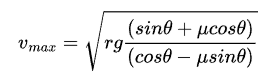if we put theta = 90 degrees in the formula above, which is the angle in the well of death, then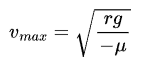But that is not acceptable, as vmax can't be an imaginary number.

I understand that if theta = 90 degrees, then the minimum value of v i.e vmin is given by: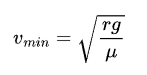This is understandable.

How do I calculate the value of vmax when theta = 90 degrees ?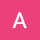ReplyForward

Last edited:
Normally, on a banked road with friction present
Check out the assumptions for 'normally'. What happens if ##v>v_{\text{max}}## ?

And what happens inif you don't substitute ##\theta ={\pi\over 2} ## but take the limit ##\theta \uparrow{\pi\over 2}## ?

 hmmm... this last one seems to be way off track... depending on ##\mu##, ##\cos\theta-\mu\sin\theta## makes ##v_{\text{max}}\uparrow\infty ## even before ##\theta ={\pi\over 2} ##. So: what's going on when ##\cos\theta-\mu\sin\theta## hits 0 ?

##\ ##

Last edited:
•topsquarkI'm assuming that what you meant to write was: what happens if v > vmax. Well, in that case, the car will start to slide up the wall of the well.
And for the 2nd part that you asked, if theta tends to pi/2, then, i'm reaching the same value for vmax as i had mentioned in the question above. Here's my calculation:

$$v_{max} = \sqrt{\frac{rg(tan\theta +\mu)}{(1 -\mu tan\theta)}}$$
$$v_{max} =\lim_{\theta \to \pi/2} \sqrt{\frac{rg(tan\theta +\mu)}{(1 -\mu tan\theta)}}$$
$$v_{max} =\sqrt{rg}\lim_{\theta \to \pi/2} \sqrt{\frac{(1 +\frac{\mu}{tan\theta}{})}{(\frac{1}{tan\theta} -\mu)}}$$
$$v_{max} =\sqrt{rg} \sqrt{\frac{1}{-\mu}}$$
$$v_{max} =\sqrt{\frac{rg}{-\mu}}$$

It's again the same result as if i had put ##\theta = \frac{\pi}{2}##.

Help is needed.

As I indicated, I was off track with that last one.

So: what's going on when ##\cos\theta-\mu\sin\theta## hits 0 ?
in other words ##\tan\theta \uparrow 1/\mu ## ?

##\ ##

what does the up arrow, between ##tan\theta## and ##\frac{1}{\mu}## signify ?

"Approaches that value ##1/\mu## from below"

"Approaches that value ##1/\mu## from below"
yes, that's the essence of the initial question that i have asked..
Somebody, kindly throw some light on this question..

yes, that's the essence of the initial question that i have asked..
So we find that ##v_\text{max}## has no upper bound for ##\theta\ge\arctan{1\over\mu}##.
I.e. the answer to your initial question is "there is no maximum".

Now all we have to sort out is what is going on physically. That involves digging into the derivation of the expression for ##v_\text{max}####\ ##

Do you want a hint ?

Homework Statement::
On a banked road with friction present, vmax is given by:
View attachment 317645

I would like you to go over the derivation of this equation. Notice that, when we are talking about the vertical "wall of death," the friction force must be acting upward. In the derivation of the equation you posted, the friction force is assumed to be down the slope. If it did that then your car will fall down the wall. So you need to reorient the frictional force to point up the slope. You get a similar equation:
##v_{max}= \sqrt{rg \dfrac{(sin( \theta ) - \mu ~ cos( \theta ) )}{( cos( \theta ) + \mu ~ sin( \theta ) )}}##
which is perfectly well-behaved for ##\theta = 90##.

-Dan

I would like you to go over the derivation of this equation. Notice that, when we are talking about the vertical "wall of death," the friction force must be acting upward. In the derivation of the equation you posted, the friction force is assumed to be down the slope. If it did that then your car will fall down the wall. So you need to reorient the frictional force to point up the slope. You get a similar equation:
##v_{max}= \sqrt{rg \dfrac{(sin( \theta ) - \mu ~ cos( \theta ) )}{( cos( \theta ) + \mu ~ sin( \theta ) )}}##
which is perfectly well-behaved for ##\theta = 90##.

-Dan
What you wrote is the equation for ##v_{min}##. The friction will not always act upward. Since we are trying to find out vmax, therefore, the friction will act downward. In my original question, i did mention that ##v_{min}## will be = ##\sqrt{\frac{rg}{\mu}}##.
The question remains as it is..

Last edited:
What you wrote is the equation for ##v_{min}##. In my original question, i did mention that ##v_{min}## will be = ##\sqrt{\frac{rg}{\mu}}##.
The question remains as it is..
Normally I'd agree with you. But in this case can the friction force be pointing downward? If ##\theta < 90## the normal force contributes to keeping the car at its height. But if the wall is vertical then N points solely in the radial direction, without any vertical component. The friction force is the only thing holding the car up.

There isn't really a "##v_{max}##" in this case.

-Dan

•NTesla, Lnewqban and 256bits
Maximum:
The maximum velocity in that case is limited only by the g-force pulling the rider or driver against the wall of the well.

Minimum:
At 90 degrees, the only lateral force on the vehicle is its own weight.
Like it happens to a car in a horizontal curve, the only way to compensate that lateral force is by steering the front wheels.

Because of the above, there is a minimum value of tangential velocity that is needed.
That forward velocity induces enough centrifugal effect to reach a minimum necessary value of normal force and lateral friction force to prevent the car from sliding down the well.

Maximum:
The maximum velocity in that case is limited only by the g-force pulling the rider or driver against the wall of the well.
What's the expression for vmax according to you..?

So we find that ##v_\text{max}## has no upper bound for ##\theta\ge\arctan{1\over\mu}##.
I.e. the answer to your initial question is "there is no maximum".

Now all we have to sort out is what is going on physically. That involves digging into the derivation of the expression for ##v_\text{max}####\ ##
I have already derived the expression for vmax, and i had posted the expression for vmax in my original question.

Normally I'd agree with you. But in this case can the friction force be pointing downward? If ##\theta < 90## the normal force contributes to keeping the car at its height. But if the wall is vertical then N points solely in the radial direction, without any vertical component. The friction force is the only thing holding the car up.

There isn't really a "##v_{max}##" in this case.

-Dan
So, if i've understood you correctly, then what you are saying is that, as long as ##\theta## is not equal to 90 degrees, friction will act downward, as we are finding vmax. But then suddenly, when ##\theta## = 90 degrees, friction will suddenly act upwards, without there being any moment when friction = 0. Friction will also act upward if velocity of car is less than vmin.

Last edited:
That link is about the topic of human endurance to high g. I'm trying to understand what will be the vmax when g = 9.8m/s^2, in a well of death. If you've figured out the expression, then kindly let me know too..

There isn't really a "##v_{max}##" in this case.
So, there wont be a vmax in 3 cases: (1) when ##\mu tan\theta## is equal to 1. (2) When ##\theta## = 90 degrees and (3) when ##\mu##=1 and ##\theta##= 45 degrees.. Is that right..?

Last edited:
So, if i've understood you correctly, then what you are saying is that, as long as ##\theta## is not equal to 90 degrees, friction will act downward, as we are finding vmax. But then suddenly, when ##\theta## = 90 degrees, friction will suddenly act upwards, without there being any moment when friction = 0. Friction will also act upward if velocity of car is less than vmin.
There is no 'suddenly'.

Check out the assumptions for 'normally'.
The expressions including ##\mu## assume a direction for the friction force. And ##\mu N## is the maximum friction force. When ##v## runs from ##v_\text{min}## to ##v_\text{max}##, the friction force varies gradually from a maximum in one direction to a maximum in the other.

##\ ##

There is no 'suddenly'.

The expressions including ##\mu## assume a direction for the friction force. And ##\mu N## is the maximum friction force. When ##v## runs from ##v_\text{min}## to ##v_\text{max}##, the friction force varies gradually from a maximum in one direction to a maximum in the other.

##\ ##
##\mu N_{max}## is the maximum friction force. Value of N will keep on varying since N is a function of velocity.. isn't it ?

That link is about the topic of human endurance to high g. I'm trying to understand what will be the vmax when g = 9.8m/s^2, in a well of death. If you've figured out the expression, then kindly let me know too..
Please, consider how heavy that person feels while doing this performance.
That is the limit to the velocity.

Please, consider how heavy that person feels while doing this performance.
That is the limit to the velocity.
let's say there isn't a person doing the round, it's just a machine.. Then what will be the vmax when theta= 90 degrees..?

let's say there isn't a person doing the round, it's just a machine.. Then what will be the vmax when theta= 90 degrees..?
The maximum speed that the machine can develop.

You are after the maximum speed with which a car can take a curve without sliding out.
Once the angle of bank grows over 45, that maximum needed speed starts to decrease as the angle increases, simply because the effective radius of the turn also starts to decrease.
Once the bank of a curve reaches 90 degrees, there is no more curve.

The shape of your road has gone from a circle to a cone to a cylinder!

maximum needed speed starts to decrease...
Shouldn't it be minimum needed speed, which according to me should continue to increase as theta increases..

Could someone please let me know if my statements in post# 19 and post#21 above, are right or wrong..

post#19: So, there wont be a vmax in 3 cases: (1) when ##\mu tan\theta## is equal to 1. (2) When ##\theta## = 90 degrees and (3) when ##\mu##=1 and ##\theta##= 45 degrees.. Is that right..?

post#21: ##\mu N_{max}## is the maximum friction force. Value of N will keep on varying since N is a function of velocity.. isn't it ?

Last edited:
Shouldn't it be minimum needed speed, which according to me should continue to increase as theta increases..
Is that a question or an assertion?
Why should it continue to increase?

Could someone please let me know if my statements in post# 19 and post#21 above, are right or wrong..

post#19: So, there wont be a vmax in 3 cases: (1) when ##\mu tan\theta## is equal to 1. (2) When ##\theta## = 90 degrees and (3) when ##\mu##=1 and ##\theta##= 45 degrees.. Is that right..?

post#21: ##\mu N_{max}## is the maximum friction force. Value of N will keep on varying since N is a function of velocity.. isn't it ?
The answer to your question is simply to sketch a FBD of the situation. If angle is less than 90 degrees there will be a minimum speed and maximum speed in order to keep the car at a specific height. The normal force has a component in the vertical direction and is a counter to the weight component down the slope.

But in this case, the wall is vertical so the normal force no longer has a component in the vertical direction. The only force that can counter the weight is friction. So friction has to be pointed upward. Technically this means that there is no ##v_{max}## because any higher speed will not change the friction situation, nor will it change anything about the height. But if the speed is too low then friction cannot support the weight of the car.

-Dan

•NTesla, nasu and Lnewqban
First, I'm not sure where you got that this equation is for ##v_{max}##; it is for ##v## in general.

Second, the 'true' equation is:
$$\frac{v^2}{rg} = \frac{\sin\theta + \frac{f}{N}\cos\theta}{\cos\theta - \frac{f}{N}\sin\theta}$$
Where ##f## is the friction pointing down the slope, which means a negative value is possible; it only means it is going against the slope.

What is the value of ##\frac{f}{N}##? Well:
$$mg = N\cos\theta - f\sin\theta$$
Therefore:
$$\frac{f}{N} = \frac{\cos\theta - \frac{mg}{N}}{\sin\theta}$$
Note how it can have a negative value. Putting it in our original equation:
$$\frac{v^2}{rg} = \frac{\sin\theta + \left(\frac{\cos\theta - \frac{mg}{N}}{\sin\theta}\right)\cos\theta}{\cos\theta - \left(\frac{\cos\theta - \frac{mg}{N}}{\sin\theta}\right)\sin\theta}$$
Or:
$$\frac{m\frac{v^2}{r}}{N} = \sin\theta +\frac{\cos\theta - \frac{mg}{N}}{\tan\theta}$$
Isolating ##N## we get either:
$$N= \frac{m\frac{v^2}{r}\tan\theta + mg}{\sin\theta \tan\theta +\cos\theta}$$
or:
$$N = \frac{m\frac{v^2}{r} + \frac{mg}{\tan\theta}}{\sin\theta + \frac{\cos\theta}{\tan\theta}}$$
• In the first form, assuming ##\theta=0##, we get ##N=mg##.
• In the second form, assuming ##\theta = 90°##, we get ##N=m\frac{v^2}{r}##.
That's it. There is no ##v_{max}##. Whether ##\theta=0## or ##\theta=90°## or anywhere in between, you can go as fast as you want in any case. Only the normal force will change, which is independent of ##v## when ##\theta=0## and independent of ##g## when ##\theta=90°##.

But let's isolate ##\frac{f}{N}## in our original equation instead:
$$\frac{f}{N} = \frac{\frac{v^2}{rg}\cos\theta - \sin\theta}{\frac{v^2}{rg}\sin\theta + \cos\theta}$$
Or:
$$\frac{f}{N} = \frac{\frac{v^2}{rg} - \tan\theta}{\frac{v^2}{rg}\tan\theta + 1}$$
And we know that to remain static (our assumption in the first place i.e. no sliding):
$$\mu_s > \left|\frac{f}{N}\right|$$
So:
$$\mu_s > \left|\frac{\frac{v^2}{rg} - \tan\theta}{\frac{v^2}{rg}\tan\theta + 1}\right|$$
But that doesn't indicate a ##v_{min}## either, just a ##\mu_{s,\ min}##. That only means that the required friction coefficient will vary between ##\frac{v^2}{rg}## at ##\theta=0## (such that the vehicle doesn't slide out the curve) and ##\frac{rg}{v^2}## at ##\theta=90°## (such that the vehicle doesn't slide down, or "into" the curve).

The only notable thing is that if ##\frac{v^2}{rg} = \tan\theta## then ##f=0## and therefore no friction is required.

We can find a ##v_{min}## and ##v_{max}## such that the vehicle doesn't begin to slide in either way.

Assuming a given ##\mu_s##, we go back to our original equation (converted to the ##\tan## equivalent):
$$\frac{v_{min}^2}{rg} = \frac{\tan\theta + (-\mu_s)}{1 - (-\mu_s)\tan\theta}$$
$$\frac{v_{max}^2}{rg} = \frac{\tan\theta + \mu_s}{1 - \mu_s\tan\theta}$$
In both cases, the right-hand side cannot be negative, so:
$$\frac{v_{min}^2}{rg} = \frac{\max\{\tan\theta; \mu_s\} - \mu_s}{1 + \mu_s\tan\theta}$$
$$\frac{v_{max}^2}{rg} = \frac{\tan\theta + \mu_s}{1 - \mu_s\min\{\tan\theta;\frac{1}{\mu_s}\}}$$
I think I got it all right.

•NTesla
Hi @NTesla. Can l put my twopence worth in?

If you go round a banked curve at a sufficiently high speed, you tend to slide upwards. (You should understand why this happens.) Static friction opposes the upwards sliding by acting downwards.

If ##\theta \lt 90^o## and you are going too fast, you will may slide upwards because downwards friction isn't big enough to prevent the sliding. Your maximum speed to avoid sliding upwards is ##V_{max}##.

Note that if ##\theta= 90^o## there is no tendency to slide upwards (and you should understand why).

The equation for ##V_{max}## applies only when the frictional force is at its maximum (limiting) value of ##\mu N## and is acting downwards - because that’s how the ##V_{max}## equation is derived. But when ##\theta = 90^0## there is no downwards friction because there is no tendency to move upwards. Friction will act upwards and it's magntiude will equal ##|mg|##; the equation for ##V_{max}## is inapplicable.
_____________

Additional note. It’s not just ##\theta = 90^o## where care is needed. For example if ##\mu = 0.9## and ##\theta = 60^o## then ##\cos(\theta) - \mu \sin(\theta) = \cos(60^o) – 0.9\sin(60^o) = -0.279## which gives a negative value inside the square root.

That’s because the ##V_{max}## equation cannot be used because friction doesn’t need to reach its limiting value to prevent upwards sliding for these values of ##\mu## and ##\theta##.

The ##V_{max}## equation is therefore inapplicable in this case- and this was signalled by the fact that ##\cos(\theta) - \mu \sin(\theta)## was negative.

Last edited:
•NTesla, MatinSAR, topsquark and 1 other person
But that is not acceptable, as vmax can't be an imaginary number.
When you write an equation to represent a physical behaviour, it often only applies in a range of situations. In this case, in writing the equation, you have assumed that there will be a speed at which it will slide up. If you get a silly answer, likely the assumption was false.
Even before you substituted θ=90° there was a problem. You can see that for any θ>0 there are values of μ which give an infinite or imaginary result, telling you it cannot slide up. What θ=90° did was to push all values of μ into that class.

•MatinSAR, topsquark and Lnewqban
This reminds me of the problem of trying to slide a box across a rough horizontal surface with a force applied at a downward angle: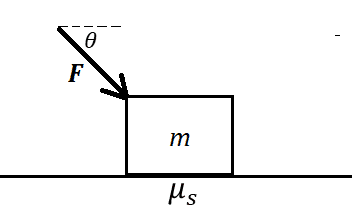For a given ##\mu_s##, if ##\theta > \tan^{-1}(1/\mu_s)##, then the box will not slide no matter how strong the force ##F##.

Likewise, for this problem. If ##\theta > \tan^{-1}(1/\mu_s)##, then the car will not slide upward on the slope no matter how fast the car is traveling. There is no ##v_{max}## for ##\theta > \tan^{-1}(1/\mu_s)##.

•Steve4Physics, MatinSAR, topsquark and 1 other person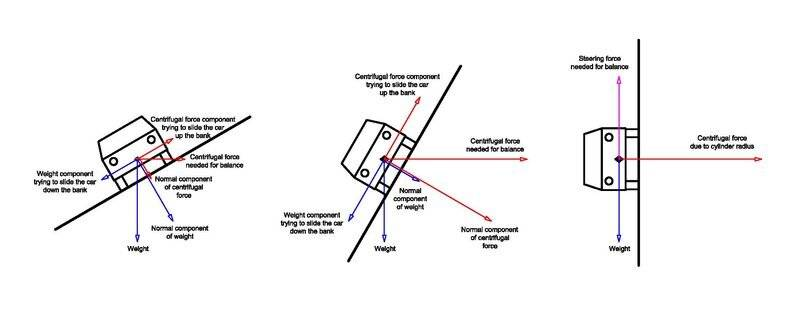#### Attachments

• Banking car.pdf
89.7 KB · Views: 44
•MatinSAR and topsquark
In the vertical wall case (horizontal rider), what force is balancing the torques about the point of contact?

P.s. This is me asking, because I’m not seeing it; not me asking the OP.

Last edited:
•NTesla and topsquark